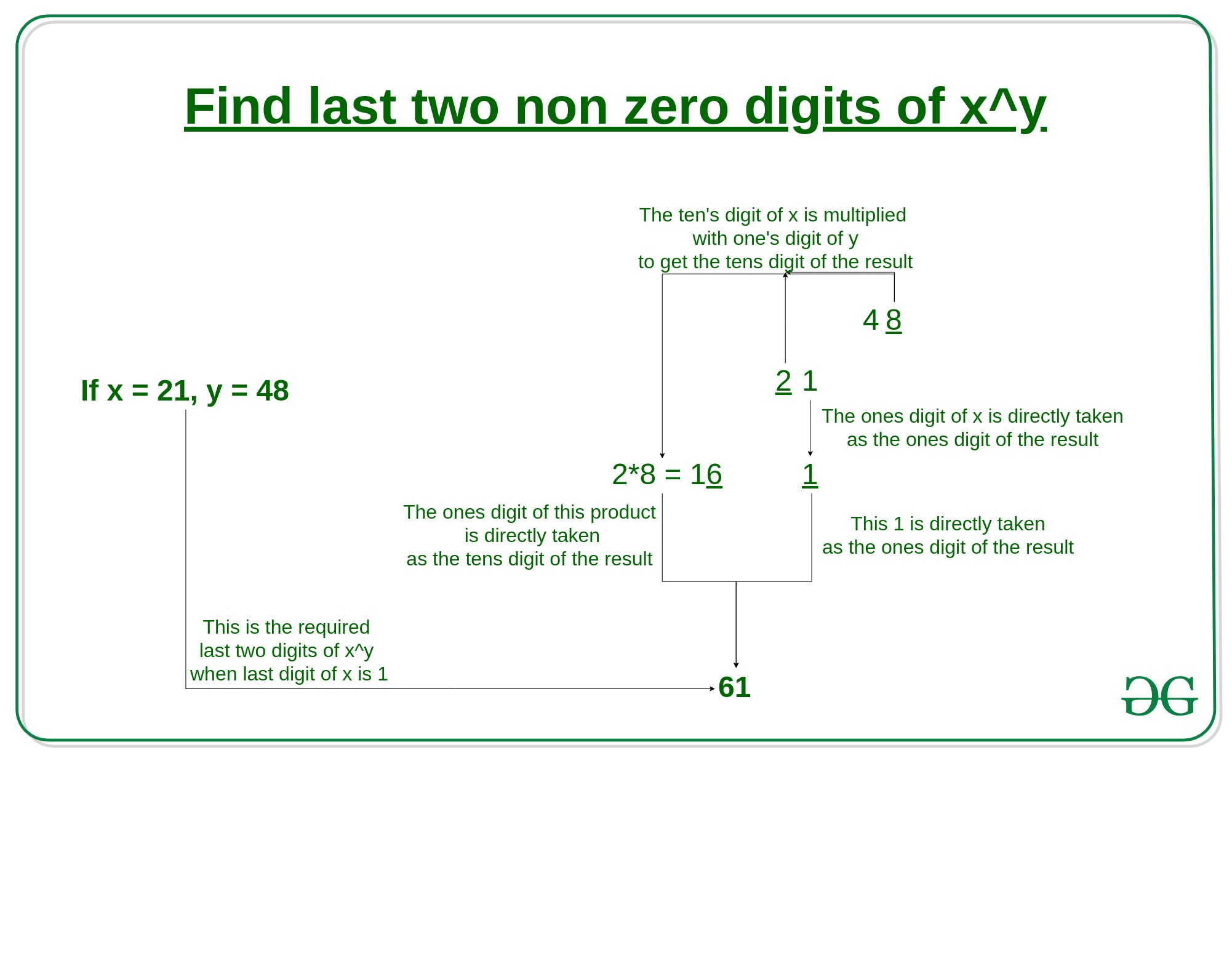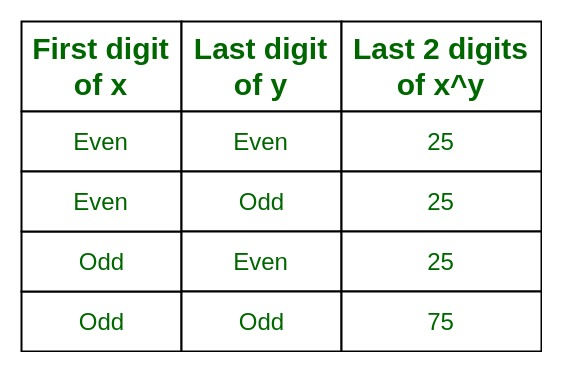# Program to find the last two digits of x^y

The task is to find the last two digits of x^y.

Since the digits with which it can end are 0-9, Hence this problem can be divided into 5 cases:

1. Case 1: when x ends with 1

For finding the last two digit of a number, when the number ends with 1 then we have to do following steps shown as in the figure.

Example: 21^48So, Last two digit of 21^48 is 81.

So, Last two digit of 31^35 is 51.

2. Case 2: when x ends with 3, 7, 9

For finding the last two digit of a number, when the number ends with 3, 7, 9 then we have to apply cyclicity concept to convert the last digit as a 1.

cyclicity of 3:

3^1 = 3
3^2 = 9
3^3 = 7
3^4 = 1

cyclicity of 7:

7^1 = 7
7^2 = 9
7^3 = 3
7^4 = 1

cyclicity of 9:

9^1 = 9
9^2 = 1

Example1: 23^34
Solution:

Example2: 37^45
Solution:

Example3: 59^22
Solution:

3. Case 3: when x ends with 2, 4, 6, 8

For finding the last digit of a number ends with 2, 4, 6, 8; We use number 76 which is a type of magic number because its square, cube and etc contain last 2 digit numbers as itself i.e 76.

Take an example :

square of 76 = 5776, its last two digit =76
cube of 76 = 438976, its last two digit=76

So we take two cases:

1. if (2^10)^even power then it always return 76 .
2. if (2^10)^odd power then it always return 24 .

Steps for finding last two digits

```
Firstly, convert given number in to these formats if  (2^10)^power.
Here power will be odd or even according to the question.
Now, check power will be odd or even.
if power is odd then its value will be 24.
if power is even then its value will be 76.
```

Examples

Example1: Find last 2 digit of 2^453.

Solution:

• Step 1:- conversion
2^453 = (2^10)^45 * 2^3
• Step 2:- odd power so we take 24
= 24 * 8
= 192

So, Last two non zero digits of 2^453 are 92.

Example2: Find last 2 digits of 4^972.

Solution:

• step 1:- conversion
4^972 = (2^2)^972
= 2^1944
= (2^10)^194 * 2^4
• step 2:- even power so, we take 76
= 76 * 16
= 1216

So, Last two non zero digits of 4^972 are 16.

Example3: Find last 2 digits of 6^600.

Solution:

• step 1:- conversion
6^600 = (2)^600 * (3)^600
= (2^10)^60 * ((3)^4)^150 {Apply case 2 in (3)^600}
• step 2:- (2^10)^60 has even power so, we take 76 as the last digit
• step 3:- Solve ((3)^4)^150, we get 01 as the last digit
• step 4:- last digit of (2^10)^60 i.e 76 multiply with the last digit of ((3)^4)^150 i.e 01
• step 5:- i.e 76 * 01 = 76

So, Last two non zero of 6^600 is 76.

Example4: Find last 2 digits of 8^330.

Solution:

• step 1:- conversion
8^33 = (2^3)^110
= (2)^330
• step 2:- (2^10)^33 has odd power so, we take 24 as the last digit

So, Last two non zero digits of 8^330 are 24.

4. Case 4: when x ends with 5

For finding the last two digit of a number, when the number ends with 5 then we have to follow the table which is given below.Example1: Find last 2 digit of 25^25.

Solution:

• first digit of number is 2 i.e even
• Last digit of a power is 5 i.e odd
• Now, even-odd combination gives last digit as a 25

So, the last two non zero digits of 25^25 are 25.

Example2: Find last 2 digit of 25^222.

Solution:

• first digit of number is 2 i.e even
• Last digit of a power is 2 i.e even
• Now, even-even combination gives last digit as a 25

So, the last two non zero digits of 25^222 are 25.

Example3: Find last 2 digit of 165^222.

Solution:

• first digit of number is 1 i.e odd
• Last digit of a power is 2 i.e even
• Now, odd-even combination gives last digit as a 25

So, the last two non zero digits of 165^222 are 25.

Example4: Find last 2 digit of 165^221.

Solution:

• first digit of number is 1 i.e odd
• Last digit of a power is 1 i.e odd
• Now, odd-odd combination gives last digit as a 75

So, the last two non zero digits of 165^221 are 75.

5. Case 5: when x ends with 0

For finding the last two digit of a number, when the number ends with 0 then we have to check next digit and according to the digit calculate the last digit.

Example: Find last 2 digit of 150^221.

Solution:

• 150 last digit is 0 so we check next digit i.e 5 and apply case 4
• first digit of number is 1 i.e odd
• Last digit of a power is 1 i.e odd
• Now, odd-odd combination gives last digit as a 75

So, the last two non zero digits of 165^221 is 75.

Attention reader! Don’t stop learning now. Get hold of all the important DSA concepts with the DSA Self Paced Course at a student-friendly price and become industry ready.

My Personal Notes arrow_drop_upCheck out this Author's contributed articles.

If you like GeeksforGeeks and would like to contribute, you can also write an article using contribute.geeksforgeeks.org or mail your article to contribute@geeksforgeeks.org. See your article appearing on the GeeksforGeeks main page and help other Geeks.

Please Improve this article if you find anything incorrect by clicking on the "Improve Article" button below.

Improved By : harshitSingh_11